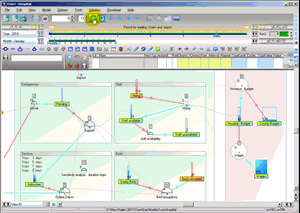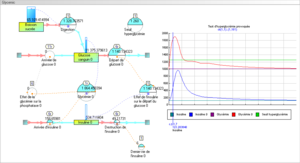Introduction The System Dynamics software TRUE, developed by True-World System Dynamics, is a tool for modeling, simulating, analyzing and optimizing multidomain dynamic applications TRUE software : applications TRUE software : principle Operation and strategic research, decision-making Conflict management dependent on scarce resources Risk Management dependent on Earth resources Macroeconomics, Microeconomics, Econometrics, Econophysic, Systemic Mathematics, traditional physics, Numerical Analysis, Statistics Relative Mechanics, Social Mathematics Accounting Medicine Statistical process control (SPC) Constraint Satisfaction Problems (CSP) Constraint programming (PPC) 4D virtual prototyping Robotics Multibody Dynamics Simulation (MDS) System dynamics modeling in discrete and continuous time (Euler) Transparent and implied mathematical modeling Coupled nonlinear differential equations of order nn because in TRUE stocks can change n times beetween two units of time differential equations of others softwares are only order n TRUE preserves the stocks integrity because in TRUE it is not possible to make unbalanced model in others softwares, it is possible to change a stock without compensation unbalanced model might create economic bubbles and financial crisis Dynamic optimization, feedback Algorithm for backtracking (BT Backtrack, loop back, dynamic backtracking) Wysiwyg graphic interface Graphical modeling, 3D modeler, bone features Real time 3D animated viewer without post-processing Multibody Dynamics Simulation Procedural Animation Example : strategic modelThe dynamic display of the variables (in a 2D or 3D view) allows to understand a model for the simulated period, that is more easily understandable than a list of static variables This model is in the True package Example : Modern Monetary TheoryPublic analysis of alternative monetary theories Working with Steve Keens Circuit Model Continuous time model by Fred Decker This model is in the True package Example : Medicine modelGlycemic control Imported Vensim (VensimPLE) model See more on ModelisationSvt This model is in the True package Example : mechanical engineering with 3D procedural animationThe motion of a non-offset piston connected to a crank through a connecting rod, can be expressed through several mathematical equations See more on wikipedia Example of piston motion This model is in the True package 3D graphic modelerThe software True can simulate a physical model and render it through a 3D animation created with its 3D modeler External 3D viewer - MDS examples 3D Pistonanimated by its physical model3D Spider made with bonesanimated by its physical modelDownload TrueRender with some examples